### IMO Shortlist 1982 problem 6

Kvaliteta:
Avg: 0.0
Težina:
Avg: 0.0
Let$S$ be a square with sides length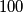$100$. Let$L$ be a path within$S$ which does not meet itself and which is composed of line segments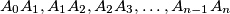$A_0A_1,A_1A_2,A_2A_3,\ldots,A_{n-1}A_n$ with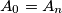$A_0=A_n$. Suppose that for every point$P$ on the boundary of$S$ there is a point of$L$ at a distance from$P$ no greater than$1\over2$. Prove that there are two points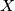$X$ and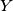$Y$ of$L$ such that the distance between$X$ and$Y$ is not greater than$1$ and the length of the part of$L$ which lies between$X$ and$Y$ is not smaller than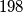$198$.
Source: Međunarodna matematička olimpijada, shortlist 1982